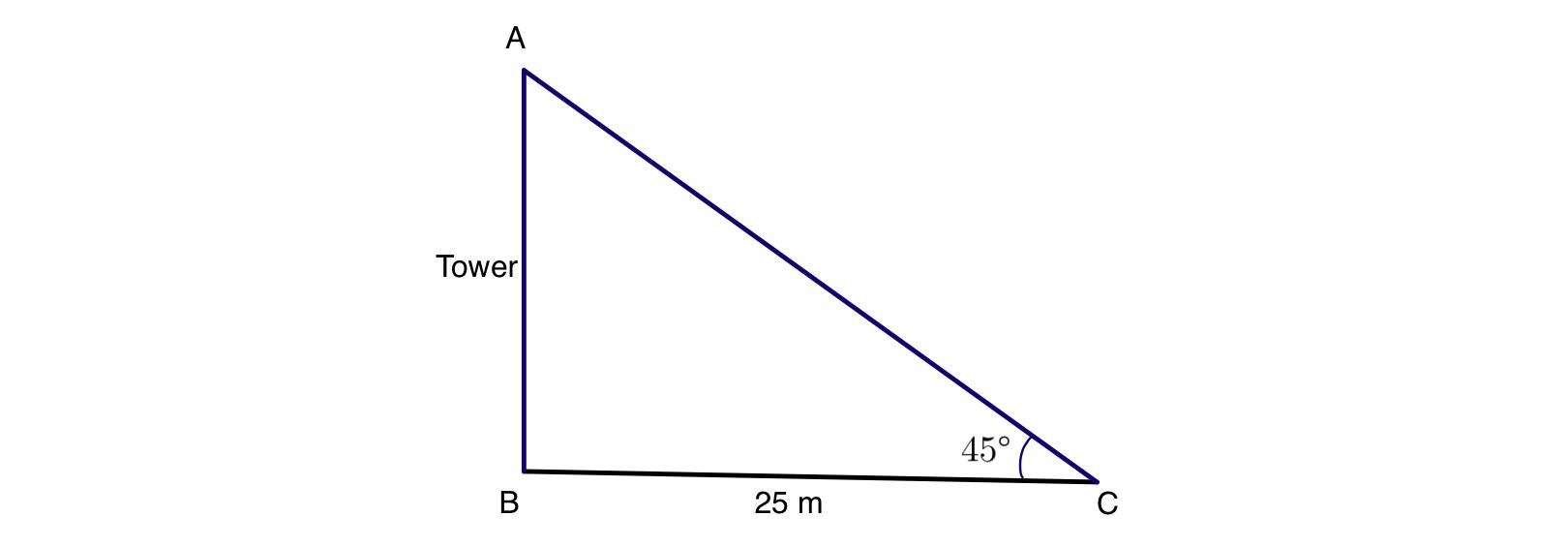QuestionAnswers

# A tower stands vertically on the ground. From a point on the ground which is 25 m away from the foot of the tower , the angle of elevation of the top of the tower is found to be $$45^{0}$$. Then the height (in meters) of the tower is:A. $$25\sqrt{2}$$B. $$25\sqrt{3}$$C. 25D. 12.5Verified
130.5k+ views
Hint: To find the solution we need to know that for a right angle triangle $$\triangle ABC$$ if $$\angle B=90^{\circ }$$ and $$\angle C=\theta$$,
then $$\tan \theta =\dfrac{\text{Perpendicular} }{\text{Base} } =\dfrac{AB}{BC}$$........(1)
So let us draw the diagram,Let us consider the height of the tower AB to be x meter.
And it is given that the distance between any point C to the foot of the tower AB is 25 m, i.e, BC = 25 m.
The angle of elevation from the point C to the top of the tower is $$45^{\circ}$$.
$$\therefore \angle C=45^{\circ }$$
Now for the right angle triangle $$\triangle ABC$$,
$$\tan \angle C=\dfrac{AB}{BC}$$ [by formula (1)]
$$\Rightarrow \tan 45^{\circ }=\dfrac{x}{25}$$
$$\Rightarrow 25\times \tan 45^{\circ }=x$$
$$\Rightarrow x=25\times \tan 45^{\circ }$$
Now as we know that the value of $$\tan 45^{\circ }$$ is 1.
So from the above equation we get,
$$x=25\times 1$$
$$\Rightarrow x=25$$

So, the correct answer is “Option C”.

Note: While solving you need to know that the adjacent side of the given angle is called base and another one is hypotenuse and the opposite side of that angle is always considered as the height of the right angle triangle. And among these three sides hypotenuse is the largest one.